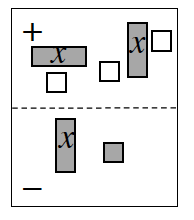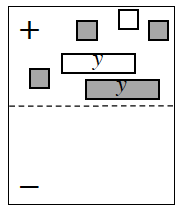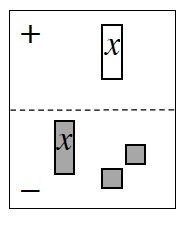### Home > CCA > Chapter A > Lesson A.1.4 > ProblemA-45

A-45.

Write and simplify the algebraic expression shown in each Expression Mat below.1.$\left(2x − 3\right) − \left( ? \right)$

1.Which tile pairs make zeros?

1.$−x −\left(x + 2\right) = −2x − 2$

Click the link at right for the full version of the eTool: A-45a HW eTool